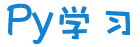注册    登录创作新主题

Python
 python开源   Django   Python   DjangoApp   pycharm
DATA
 docker   Elasticsearch

 问与答   闲聊   招聘   翻译   创业   分享发现   分享创造   求职   区块链   支付之战
aigc
 aigc   chatgpt
WEB开发
 linux   MongoDB   Redis   DATABASE   NGINX   其他Web框架   web工具   zookeeper   tornado   NoSql   Bootstrap   js   peewee   Git   bottle   IE   MQ   Jquery

Python88.com
 反馈   公告   社区推广

Py学习  »  机器学习算法

# 机器学习 11 种特征选择策略总结！`本文与你分享可应用于特征选择的各种技术的有用指南。`

“特征选择”意味着可以保留一些特征并放弃其他一些特征。本文的目的是概述一些特征选择策略：

1. 删除未使用的列
2. 删除具有缺失值的列
3. 不相关的特征
4. 低方差特征
5. 多重共线性
6. 特征系数
7. p 值
8. 方差膨胀因子 (VIF)
9. 基于特征重要性的特征选择
10. 使用 sci-kit learn 进行自动特征选择
11. 主成分分析 (PCA)

``import pandas as pddata = 'https://raw.githubusercontent.com/pycaret/pycaret/master/datasets/automobile.csv'df = pd.read_csv(data)df.sample(5)``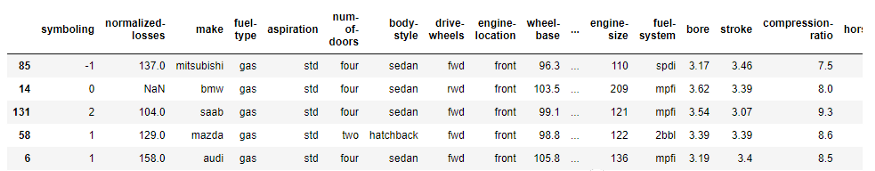``df.columns>> Index(['symboling', 'normalized-losses', 'make', 'fuel-type', 'aspiration', 'num-of-doors', 'body-style', 'drive-wheels', 'engine-location','wheel-base', 'length', 'width', 'height', 'curb-weight', 'engine-type', 'num-of-cylinders', 'engine-size', 'fuel-system', 'bore', 'stroke', 'compression-ratio', 'horsepower', 'peak-rpm', 'city-mpg', 'highway-mpg', 'price'], dtype='object')``

## 2.删除具有缺失值的列

`# total null values per columndf.isnull().sum()>>symboling             0normalized-losses   35make                 0fuel-type             0aspiration           0num-of-doors         2body-style           0drive-wheels         0engine-location       0wheel-base           0length               0width                 0height               0curb-weight           0engine-type           0num-of-cylinders     0engine-size           0fuel-system           0bore                 0stroke               0compression-ratio     0horsepower           0peak-rpm             0city-mpg             0highway-mpg           0price                 0dtype: int64`

## 3.不相关的特征

``# correlation between target and features(df.corr().loc['price'].plot(kind='barh', figsize=(4,10)))``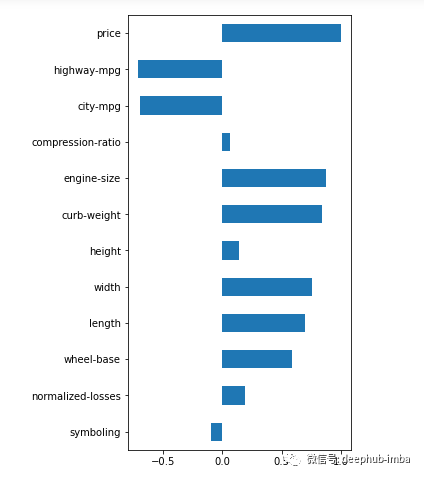``# drop uncorrelated numeric features (threshold <0.2)corr = abs(df.corr().loc['price'])corr = corr[corr<0.2]cols_to_drop = corr.index.to_list()df = df.drop(cols_to_drop, axis=1)``

```

`import seaborn as snssns.boxplot(y = 'price', x = 'fuel-type', data=df)````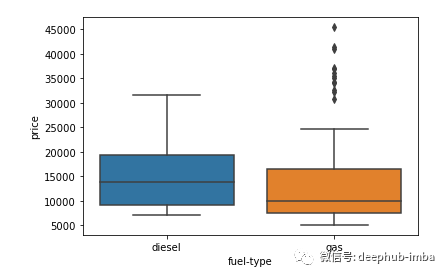## 4.低方差特征

``import numpy as np# variance of numeric features(df.select_dtypes(include=np.number).var().astype('str'))``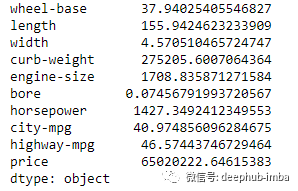``df['bore'].describe()``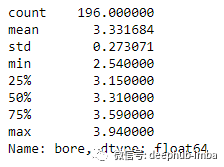## 5.多重共线性

Heatmap 是检查和寻找相关特征的最简单方法。

``import matplotlib.pyplot as pltsns.set(rc={'figure.figsize':(16,10)})sns.heatmap(df.corr(),          annot=True,          linewidths=.5,          center=0,          cbar=False,          cmap="PiYG")plt.show()``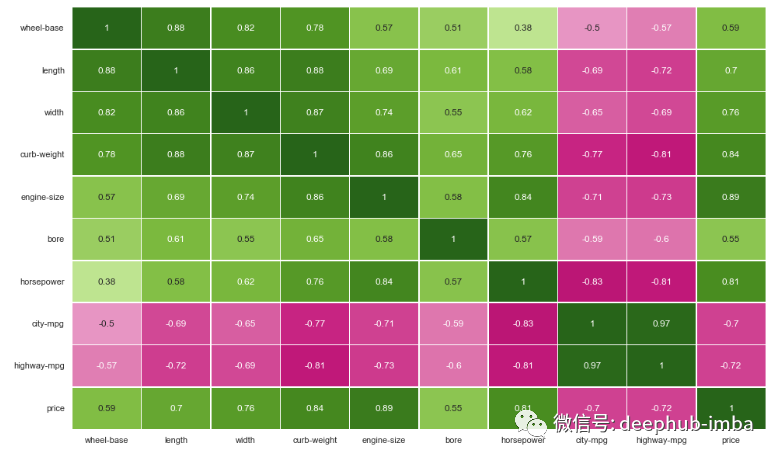``# drop correlated featuresdf = df.drop(['length', 'width', 'curb-weight', 'engine-size', 'city-mpg'], axis=1)``

``df_cat = df[['fuel-type', 'body-style']]df_cat.sample(5)``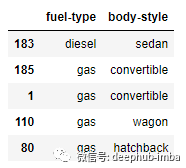``crosstab = pd.crosstab(df_cat['fuel-type'], df_cat['body-style'])crosstab``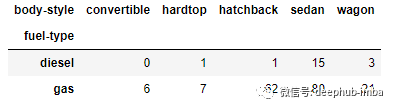``from scipy.stats import chi2_contingencychi2_contingency(crosstab)``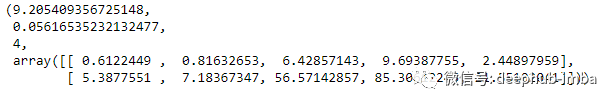p 值 <0.05，因此我们可以拒绝特征之间没有关联的原假设，即两个特征之间存在统计上显着的关系。

``# drop columns with missing valuesdf = df.dropna()from sklearn.model_selection import train_test_split# get dummies for categorical featuresdf = pd.get_dummies(df, drop_first=True)# X featuresX = df.drop('price', axis=1)# y targety = df['price']# split data into training and testing setX_train, X_test, y_train, y_test = train_test_split(X, y, test_size=0.3, random_state=42)from sklearn.linear_model import LinearRegression# scalingfrom sklearn.preprocessing import StandardScalerscaler = StandardScaler()X_train = scaler.fit_transform(X_train)X_test = scaler.fit_transform(X_test)# convert back to dataframeX_train = pd.DataFrame(X_train, columns = X.columns.to_list())X_test = pd.DataFrame(X_test, columns = X.columns.to_list())# instantiate modelmodel = LinearRegression()# fitmodel.fit(X_train, y_train)``

## 6.特征系数

``# feature coefficientscoeffs = model.coef_# visualizing coefficientsindex = X_train.columns.tolist()(pd.DataFrame(coeffs, index = index, columns = ['coeff']).sort_values(by = 'coeff').plot(kind='barh', figsize=(4,10)))``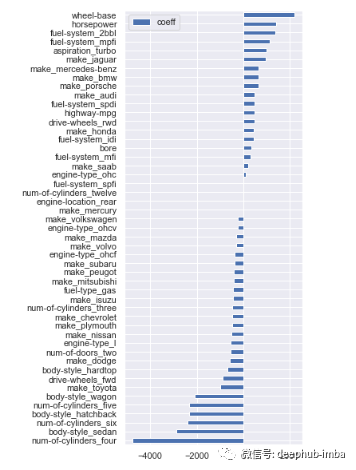``# filter variables near zero coefficient valuetemp = pd.DataFrame(coeffs, index = index, columns = ['coeff']).sort_values(by = 'coeff')temp = temp[(temp['coeff']>1) | (temp['coeff']# drop those featurescols_coeff = temp.index.to_list()X_train = X_train[cols_coeff]X_test = X_test[cols_coeff]``

## 7.p 值

``import statsmodels.api as smols = sm.OLS(y, X).fit()print(ols.summary())``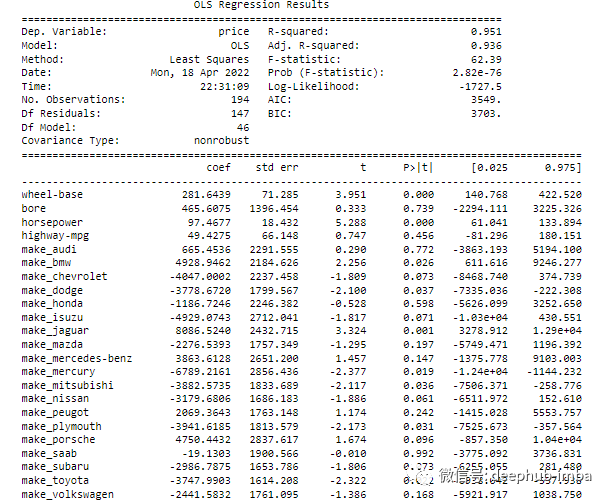## 8.方差膨胀因子 (VIF)

• VIF = 1 表示无相关性
• VIF = 1-5 中等相关性
• VIF >5 高相关

VIF 是一种消除多重共线性特征的有用技术。对于我们的演示，将所有 VIF 高于10的删除。

``from statsmodels.stats.outliers_influence import variance_inflation_factor# calculate VIFvif = pd.Series([variance_inflation_factor(X.values, i) for i in range(X.shape)], index=X.columns)# display VIFs in a tableindex = X_train.columns.tolist()vif_df = pd.DataFrame(vif, index = index, columns = ['vif']).sort_values(by = 'vif', ascending=False)vif_df[vif_df['vif']<10]``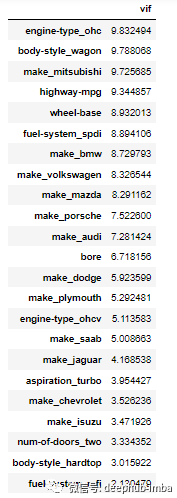## 9.基于特征重要性选择

``from sklearn.ensemble import RandomForestClassifier# instantiate modelmodel = RandomForestClassifier(n_estimators=200, random_state=0)# fit modelmodel.fit(X,y)``

``# feature importanceimportances = model.feature_importances_# visualizationcols = X.columns(pd.DataFrame(importances, cols, columns = ['importance']).sort_values(by='importance', ascending=True).plot(kind='barh', figsize=(4,10)))``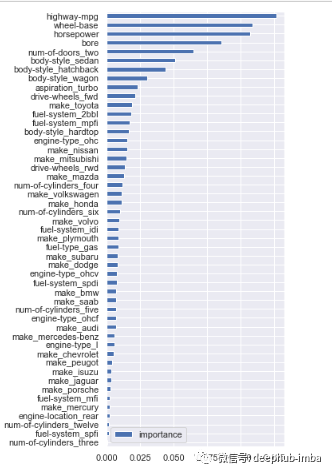``# calculate standard deviation of feature importancesstd = np.std([i.feature_importances_ for i in model.estimators_], axis=0)# visualizationfeat_with_importance = pd.Series(importances, X.columns)fig, ax = plt.subplots(figsize=(12,5))feat_with_importance.plot.bar(yerr=std, ax=ax)ax.set_title("Feature importances")ax.set_ylabel("Mean decrease in impurity")``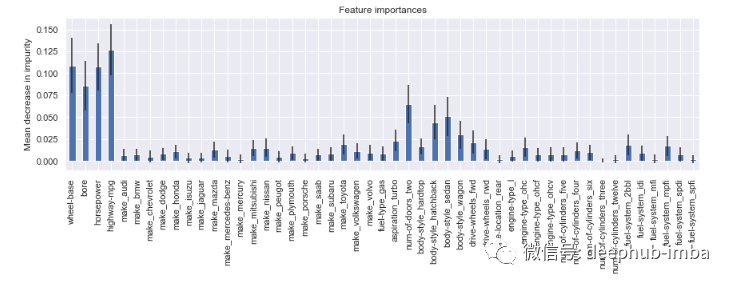## ‍10.使用 Scikit Learn 自动选择特征‍

sklearn 库中有一个完整的模块，只需几行代码即可处理特征选择。

sklearn 中有许多自动化流程，但这里我只展示一些：

``# import modulesfrom sklearn.feature_selection import (SelectKBest, chi2, SelectPercentile, SelectFromModel, SequentialFeatureSelector, SequentialFeatureSelector)``

``# select K best featuresX_best = SelectKBest(chi2, k=10).fit_transform(X,y)# number of best featuresX_best.shape>> 10``
```‍

```

``# keep 75% top featuresX_top = SelectPercentile(chi2, percentile = 75).fit_transform(X,y)# number of best featuresX_top.shape>> 36``

``# implement algorithmfrom sklearn.svm import LinearSVCmodel = LinearSVC(penalty= 'l1', C = 0.002, dual=False)model.fit(X,y)# select features using the meta transformerselector = SelectFromModel(estimator = model, prefit=True)X_new = selector.transform(X)X_new.shape>> 2# names of selected featuresfeature_names = np.array(X.columns)feature_names[selector.get_support()]>> array(['wheel-base', 'horsepower'], dtype=object)``
`‍`

``# instantiate modelmodel = RandomForestClassifier(n_estimators=100, random_state=0)# select featuresselector = SequentialFeatureSelector(estimator=model, n_features_to_select=10, direction='backward', cv=2)selector.fit_transform(X,y)# check names of features selectedfeature_names = np.array(X.columns)feature_names[selector.get_support()]>> array(['bore', 'make_mitsubishi', 'make_nissan', 'make_saab',      'aspiration_turbo', 'num-of-doors_two', 'body style_hatchback', 'engine-type_ohc', 'num-of-cylinders_twelve', 'fuel-system_spdi'], dtype=object)``

11.主成分分析 (PCA)

PCA的主要目的是降低高维特征空间的维数。原始特征被重新投影到新的维度（即主成分）。最终目标是找到最能解释数据方差的特征数量。

``````# import PCA modulefrom sklearn.decomposition import PCA# scaling dataX_scaled = scaler.fit_transform(X)# fit PCA to datapca = PCA()

pca.fit(X_scaled)evr = pca.explained_variance_ratio_# visualizing the variance explained by each principal componentsplt.figure(figsize=(12, 5))plt.plot(range(0, len(evr)), evr.cumsum(), marker="o", linestyle="--")plt.xlabel("Number of components")plt.ylabel("Cumulative explained variance")``````20 个主成分解释了超过 80% 的方差，因此可以将模型拟合到这 20 个成分（特征）。可以预先确定方差阈值并选择所需的主成分数量。

## 总结

（版权归原作者所有，侵删）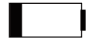Python社区是高质量的Python/Django开发社区